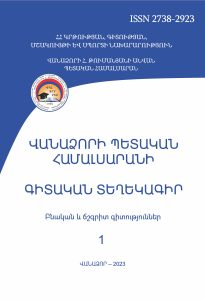# Coding Application

Scientific Proceedings of  Vanadzor State University Natural and Exact Sciences (ISSN 2738-2923)

2023 vol 1

Coding Application

Suren Eksuzyan

SummaryKey words: message code, coding equivalence, subset code, discrete optimization problem, set partitioning, knapsack problem, variant observation algorithm

The work is devoted to the development of the algorithm for observing all subsets of the set.

For that purpose, coding for subsets of the set is considered, in such a way as to create a 1-1 correspondence between the set of subsets of the set and the set of codes.

A convenient arrangement of the observation of all those codes allows the observation of all subsets of the set in a convenient way.

Subsets of a set are coded in the binary number system (with binary numbers). The problem of considering subsets of a set arises when solving the knapsack problem, set partitioning problems, and other problems.

To the mentioned general problems are included a number of specific problems arising in many fields.

In the work, an algorithm for observing all subsets of the set is developed, which is suitable for solving all the mentioned problems.

The developed algorithm can be applied to the optimal solution of non-large NP-complete problems. All the known optimal solution algorithms for solving large-scale problems run in practically unacceptable time (have very long execution time). As a consequence, for the solution of large NP-complete problems, approximate algorithms with polynomial time complexity are developed, by means of which approximate solutions of the problem are obtained.

PDF